Next: Informal presentation of the Up: The Puzzle of Unfaithful Previous: The Puzzle of Unfaithful

## Knowledge set and knowledge base

Let L be any language. We will make the notion of the totality of one's knowledge explicit by the following definitions:

Definition.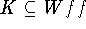is a knowledge set for St if K satisfies the following conditions:

(KS1) K is consistent.

(KS2)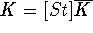, where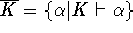.

(KS3) If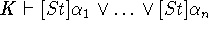then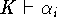for some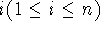.

Definition.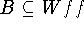is a knowledge base for St if B satisfies the following conditions:

(KB1) B is consistent.

(KB2)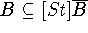, where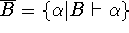,

(KB3) If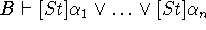then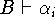for some.

By (KS2) (or (KB2)) we see that any element in K (or B, esp.) has the form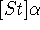. It is easy to see that if B is a knowledge base for St then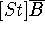is a knowledge set for St.

Let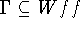be consistent. We compare the following three conditions.

1. If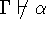then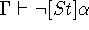.
2. If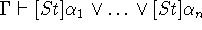then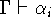for some.
3. If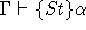then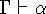or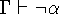.

Then we have the following

Lemma 5. (1), (2) and (3) are equivalent.

We now study the semantical characterization of knowledge sets. Let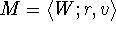be any KT5-model. For any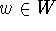and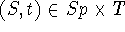, we define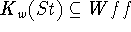by: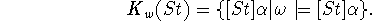Since, as we will see below,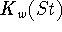is a knowledge set for St, we call it the knowledge set for St at w.

Lemma 6.is a knowledge set for St.

Let K be a knowledge set for St. We say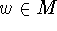characterizes K if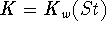.

Theorem 7. Any knowledge set is characterizable.

Yasuko Kitajima
Fri Jun 20 13:39:43 PDT 1997